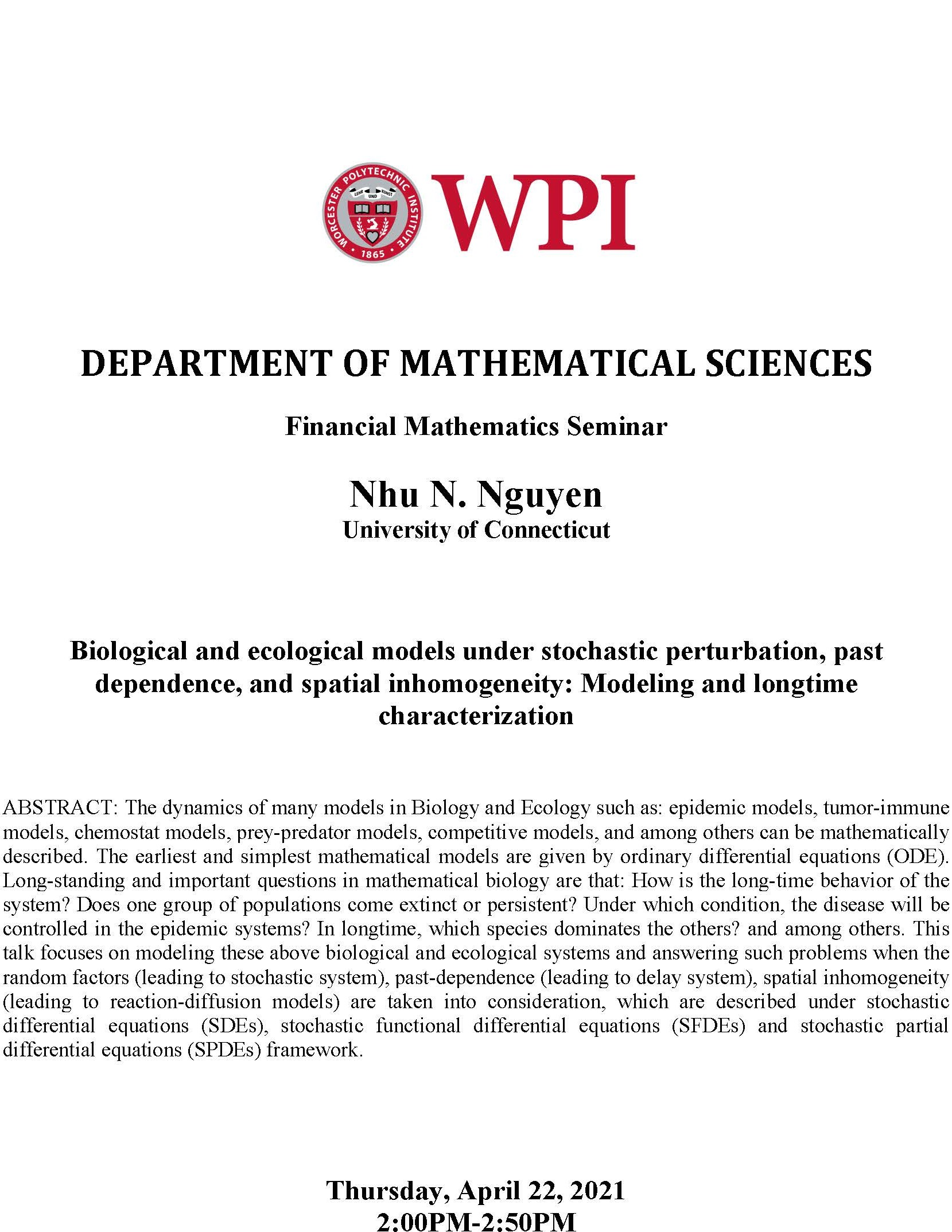# Mathematical Sciences-Financial Math Seminar - Nhu N. Nguyen (University of Conn.) "Biological and ecological models under stochastic perturbation, past dependence and spatial inhomogeneity: Modeling and longtime characterization"

## Financial Mathematics Seminar Nhu N. Nguyen- April 22, 2021.jpgThursday, April 22, 2021
2:00 pm to 2:50 pm
Floor/Room #:
via Zoom

Speaker: Nhu N. Nguyen (University of Connecticut)
Title: Biological and ecological models under stochastic perturbation, past dependence and spatial inhomogeneity: Modeling and longtime characterization

Abstract: The dynamics of many models in Biology and Ecology such as: epidemic models, tumor-immune models, chemostat models, prey-predator models, competitive models, and among others can be mathematically described. The earliest and simplest mathematical models are given by ordinary differential equations (ODE). Long-standing and important questions in mathematical biology are that: How is the long-time behavior of the system? Does one group of populations come extinct or persistent? Under which condition, the disease will be controlled in the epidemic systems?. In longtime, which species dominates the others? and among others. This talk focuses on modeling these above biological and ecological systems and answering such problems when the random factors (leading to stochastic system), past-dependence (leading to delay system), spatial inhomogeneity (leading to reaction-diffusion models) are taken into consideration, which are described under stochastic differential equations (SDEs), stochastic functional differential equations (SFDEs) and stochastic partial differential equations (SPDEs) framework.